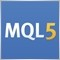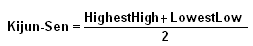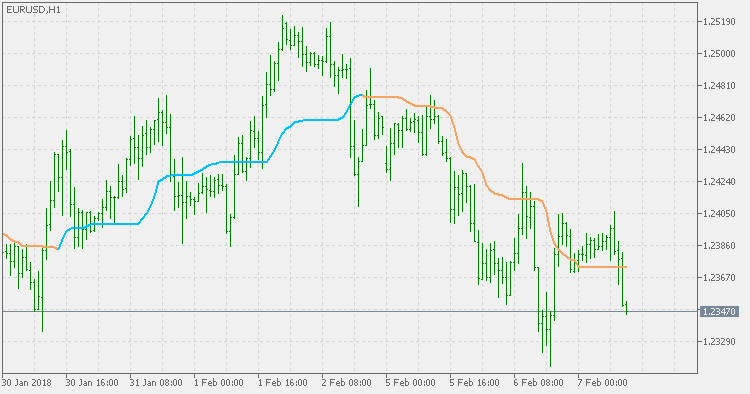# Indicators: Smoothed Kijun-Sen146198

The Kijun-Sen is a major indicator line and component of the Ichimoku Kinko Hyo indicator, also known as the Ichimoku cloud. It is generally used as a metric for medium-term momentum. The Kijun-Sen line calculation utilizes the following formula:The formula used to calculate the Kijun-Sen is almost identical to that of the formula used to calculate the Tenkan-Sen line. It differs in that the quantity of time periods used in the calculation is increased so long-term momentum can more effectively be gauged.

This version of Kijun-Sen adds one option not known in the original Kijun-Sen indicator - smoothing. The smoothing is done not by smoothing the result, but by smoothing the prices prior to being used for calculation. Hence the lag is lessened and the result is still smoother. That way some possible fake signals are filtered out.PS: in order to have the original Kijun-Sen value, set the smoothing period to less than or equal to 1. In that case, no smoothing is performed, and the values are the same as the original Kijun-Sen.